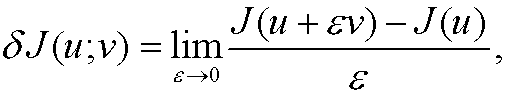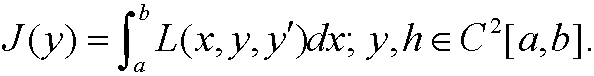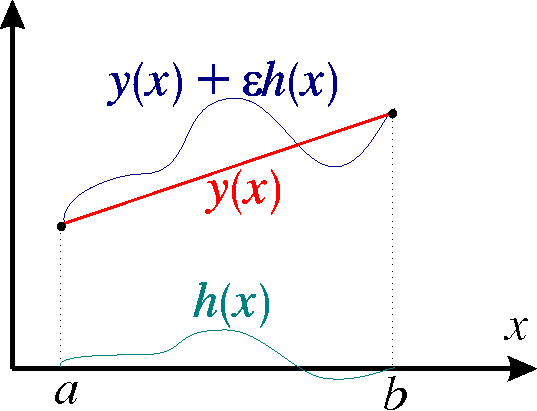# 13. Differentiation of functionals.

If u and v are elements in the vector space X we define the Gâteau-variation of the functional J(u) in the direction v according tosupposed that this limit exists.

Remark: This means thatThe increment eh is called the variation of the function y0.

Exampel 23: Suppose that J in this case is a real valued function f in C1[a,b] defined for x in Rn. If y is a unit vector in Rn  thenthe direction derivative to f in the direction y. By making a taylorexpansion we see thatExample 24: (Compare theorem 1 and the proof in part 8). Consider the functionalThenGeometrical interpretation of competing curves: